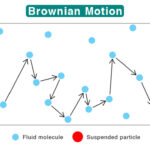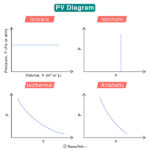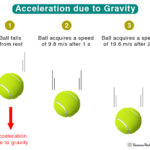Home / Physics / Terminal Velocity

# Terminal Velocity

Terminal velocity is the constant speed an object acquires after falling through fluid, like air. It occurs when the sum of the buoyant force and the drag force equals the force due to gravity. The terminal velocity is the highest velocity during the object’s fall. Since its speed is constant, the net force acting on the object is zero, and it will have no acceleration. Hence, terminal velocity is an example of balanced force.

## How is Terminal Velocity Reached

To understand more about terminal velocity, let us consider an object falling through the sky and make the following observations.

1. A force acts upon the object due to gravity, which causes it to accelerate. It means that the speed increases as the object continue to fall further.
2. The air offers resistance to the object. The air resistance is known as drag force and is proportional to the object’s speed. Thus, the drag force increases for a free-falling object.
3. Eventually, the drag force becomes equal to the force due to gravity. At this moment, there is no unbalanced force on the object. The object will no longer slow down or speed up. Rather, it will continue to fall at a constant velocity, known as the terminal velocity.

## Equation

As discussed in our article on drag force, the following equation gives its magnitude:

$F_D = \frac{1}{2} C\rho_{f} A v^2$

Where,

C : drag coefficient. It is dimensionless with values depending on the shape of the object. (For example, C = 0.47 for a sphere and C = 0.82 for a long cylinder)

ρf : density of the fluid

v : object’s velocity

A : projected cross-sectional area of the object in a direction perpendicular to the object’s motion.

At terminal velocity, v is replaced by vT. The drag force becomes

$F_D = \frac{1}{2} C\rho_{f} A v_{T}^2$

The force due to gravity is

$F = mg$

Where,

m : mass of the object

Since the object moves with a constant velocity, no net force is acting on it. This condition is only possible when the drag force is equal to the force due to gravity.

$\frac{1}{2} C\rho_{f} A v_{T}^2 = mg$
$\Rightarrow v_{T} = \sqrt{\frac{2mg}{C\rho_f A}}$

Unit: meters per second (m/s) or miles per hour (mph)

### Buoyancy Effects

When the object moves through a liquid, Archimedes Principle and buoyancy play a key role in determining its terminal velocity. The expression for terminal velocity is modified by replacing the object’s mass with its reduced mass (m – ρoV).

$v_{T} = \sqrt{\frac{2(m-\rho_o V)g}{C\rho_f A}}$

Where ρo is the density of the object.

### Viscous Fluid

If the object moves through a viscous fluid, it experiences drag force due to viscosity. According to Stokes’ law, the drag force experienced by a spherical particle is

$F_d = 6\pi \space \eta \space r \space v$

Where,

Fd : drag force

η : viscosity

r : radius of the particle

v : velocity of the particle relative to the fluid

The object is subjected to drag, buoyant, and gravitational forces at any given time. The following equation gives its terminal velocity:

$v_T = \frac{2}{9} \frac{(\rho_o – \rho_f ) g r^2}{\eta }$

## FAQs

Q.1. What is the difference between terminal velocity and free fall?

Ans. During a free fall, the only force acting on an object is the force of gravity. On the other hand, terminal velocity is calculated when no net force is acting on the object.

Q.2. How long does it take to reach terminal velocity?

Ans. The average time taken by human skydiving with a belly-down position is 12 seconds.

Q.3. What is the terminal velocity of a bullet?

Ans. Experiments have shown that bullets can have a terminal velocity of 60 to 90 m/s.

Q.4. What is the terminal velocity of a raindrop?

Ans. The maximum terminal velocity of a raindrop is 10 m/s.

Q.5. What is the terminal velocity of a 10 lb bowling ball?

Ans. The terminal velocity of a 10 lb bowling ball is 62.3 m/s.

Article was last reviewed on Thursday, September 22, 2022

### Related articlesBrownian MotionPV DiagramVelocity vs AccelerationAcceleration due to Gravity